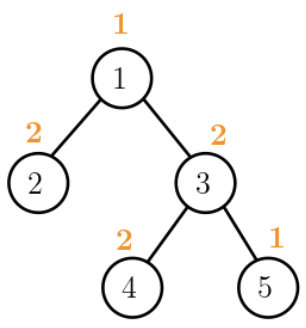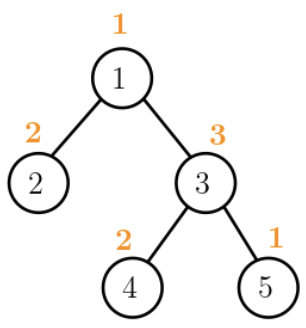시간 제한메모리 제한제출정답맞힌 사람정답 비율
2 초 (추가 시간 없음) 1024 MB47191341.935%

## 문제

A group of scientists analyzed an ant nest where several ant colonies live. They found that the ant nest is a tree structure in which each node represents the physical place where an ant colony lives, and each edge represents a tunnel connecting two ant colonies. The most interesting thing is that each colony has exactly one color and it sometimes changes its color. The color change mechanism depends on the closest pair of colonies with a certain color $c$ among the colonies lying on the path between two given colonies $A$ and $B$. The distance between two colonies is the number of tunnels of the path connecting them, that is, the number of edges of the path connecting two corresponding nodes in the tree structure.

For example, Figure A.1 (a) shows a tree structure with five ant colonies numbered 1 to 5 of colors 1, 2, 2, 2, 1, labelled in orange above the colonies, in order from colony 1 to colony 5, respectively. For color 2 and two colonies 2 and 5, the closest pair of colonies with color 2 on the path between colony 2 and colony 5 is the pair (colony 2, colony 3). But for colony 2 and colony 4, the closest pair with color 2 is the pair (colony 3, colony 4).

Suppose now that the current color 2 of colony 3 changes to color 3 as shown in Figure A.1 (b). Then there is no closest pair of colonies with color 2 on the path between colony 2 and colony 5 because only one colony has color 2. The closest pair with color 2 for colony 2 and colony 4 becomes (colony 2, colony 4).

Given colors of ant colonies, a tree structure of the ant nest, and an ordered list of update commands for the color change and query commands for the closest pair, write a program to find the closest pair of colonies with color $c$ between the two colonies $A$ and $B$ for each query $(A, B, c)$.(a) (b)

Figure A.1 An ant nest with five colonies. The numbers in orange represent colony colors.

## 입력

Your program is to read from standard input. The input starts with a line containing two integers, $n$ and $a$ ($2 ≤ n ≤ 100,000$, $2 ≤ q ≤ 100,000$), where $n$ is the number of ant colonies and $q$ is the number of update and query commands. Ant colonies are numbered from $1$ to $n$, and colors are identified with integers from $\{1, 2, \cdots , n\}$. The next line consists of $n$ positive integers representing colors for ant colonies, in order from colony $1$ to colony $n$. In the following $n-1$ lines, the $i$-th line contains a pair of integers $a_i$, $b_i$ ($1 ≤ a_i , b_i ≤ n$, $a_i ≠ b_i$) specifying the numbers of two ant colonies connected by a tunnel, which corresponds to an edge in the tree structure. In the following $q$ lines, the $i$-th line has a form of $(S, A, c)$ or $(S, A, B, c)$, where $S$ is a single uppercase character either ‘U’ or ‘Q’, representing the update and the query, respectively. In the case that $S =$ U, it has the form of $(S, A, c)$ which is an update command to change (update) the current color of colony $A$ to color $c$ ($1 ≤ A, c ≤ n$). In the case of $S =$ Q, it has the form of $(S, A, B, c)$ which is a query command to output the distance of the closest pair of colonies with color $c$ on the path between colony $A$ and colony $B$ ($1 ≤ A, B, c ≤ n$). These commands must be executed in the order given in the input.

## 출력

Your program is to write to standard output. For every query $(S, A, B, c)$ with $S =$ Q, print exactly one line containing the distance of the closest pair of colonies with color $c$ on the path between colonies $A$ and $B$ under the current status of the ant nest. If there is no pair with color $c$ between them, print -1.

## 예제 입력 1

5 5
1 2 2 2 1
1 2
3 1
3 4
3 5
Q 2 5 2
Q 2 4 2
U 3 3
Q 2 5 2
Q 2 4 2


## 예제 출력 1

2
1
-1
3


## 예제 입력 2

4 6
2 1 1 1
1 2
1 3
1 4
Q 2 3 1
Q 2 4 1
Q 3 4 1
U 1 1
Q 2 3 1
Q 2 4 1


## 예제 출력 2

2
2
2
1
1


## 힌트

The first sample corresponds to Figure A.1.